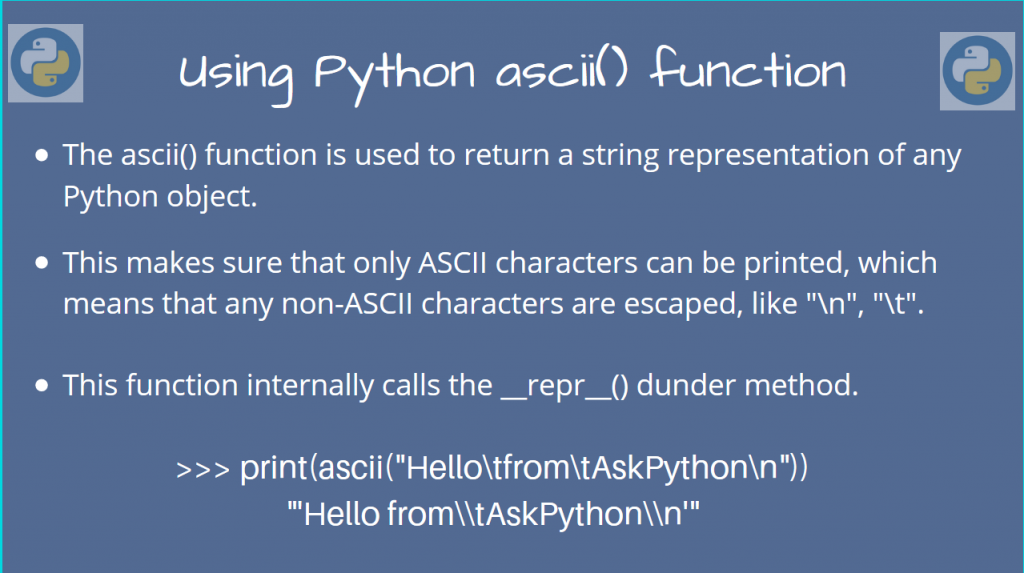# How to use the Python ascii() functionIn this article, we’ll take a look at the Python ascii() function.

The ascii() function returns a string representation of the object but only having ASCII characters as it is.

The remaining non-ASCII characters will be escaped with a backslash (\). For example, the newline character (`\n`) is a non-ASCII character.

We’ll now look at some examples to understand how it exactly works!

## Using the Python ascii() function – Some examples

The Python ascii() function takes a single argument, which can be any object. So all kinds of objects, like lists, strings, etc, are valid. This will return a string.

If you’re using it on a List, or any collection, this function will get called for each member of the collection.

Let’s take a look at this now.

### Using Python ascii() on primitive datatypes

For basic datatypes like `boolean`, `string`, `int`, they work as you expect.

```i = 15
print(ascii(i))

b = True
print(ascii(b))

s = 'abc'
print(ascii(s))

print(ascii(s))
```

Output

```'15'
'True'
"'abc'"
```

As you can see, for the non-ASCII characters (\t, \n), the backslash itself needs to be escaped.

### Using ascii() on Iterables/Collections

In case you want to use it on a list/tuple/dictionary, you still can! But, this will simply apply it to every member in the collection/iterable.

So if a list has `n` elements, we’ll get the function applied to all `n` of them, and get back a list of strings.

```m = ["Hello from AskPython 22", "AskPythön", "Hi"]
print(ascii(m))
```

Output

```['Hello from AskPython 22', 'AskPyth\xf6n', 'Hi']
```

Similarly, with a Dictionary {`key`:`value`}, it’ll be applied to both `key` and `value`.

```d = {'â':'å', '2':2, 'ç':'ć'}
print(ascii(d))
```

Output

```{'\xe2': '\xe5', '2': 2, '\xe7': '\u0107'}
```

For a tuple, it is similar to that of a list. All elements will be converted to a string representation of ASCII-like characters.

```t = ("Hellö", 123, ["AskPython"])
print(ascii(t))
```

Output

```('Hell\xf6', 123, ['AskPython'])
```

### Comparison with the repr() function

The `repr()` function is also used to return a string representation of objects. But the difference is that `repr()` prints the non-ascii characters as such.

For custom objects, the `ascii()` function internally calls the `__repr__()` function, but makes sure to escape non-ASCII characters.

Let’s experiment with this, by creating our own object, using a class.

```class MyClass:
def __init__(self, name):
self.name = name
```

Now, let’s create an object and try to invoke `ascii()` and `repr()` on it,

```my_obj = MyClass("AskPythön")
print(ascii(my_obj))
print(repr(my_obj))
```

Output

```'<__main__.MyClass object at 0x7f6adcf30940>'
```

We don’t have a `repr()` function for this class, so the default `object` definition is used. That’s why you see `MyClass object` in the output.

To change this, we must overload the `__repr__()` dunder method ourselves.

```class MyClass:
def __init__(self, name):
self.name = name
def __repr__(self):
return self.name
```

Now, when you invoke `ascii()` or `repr()`, we can directly get the name attribute!

```my_obj = MyClass("AskPythön")
print(ascii(my_obj))
print(repr(my_obj))
```

Output

```AskPyth\xf6n
In this article, we learned about using the `ascii()` function in Python and learned to use it on different types of objects.